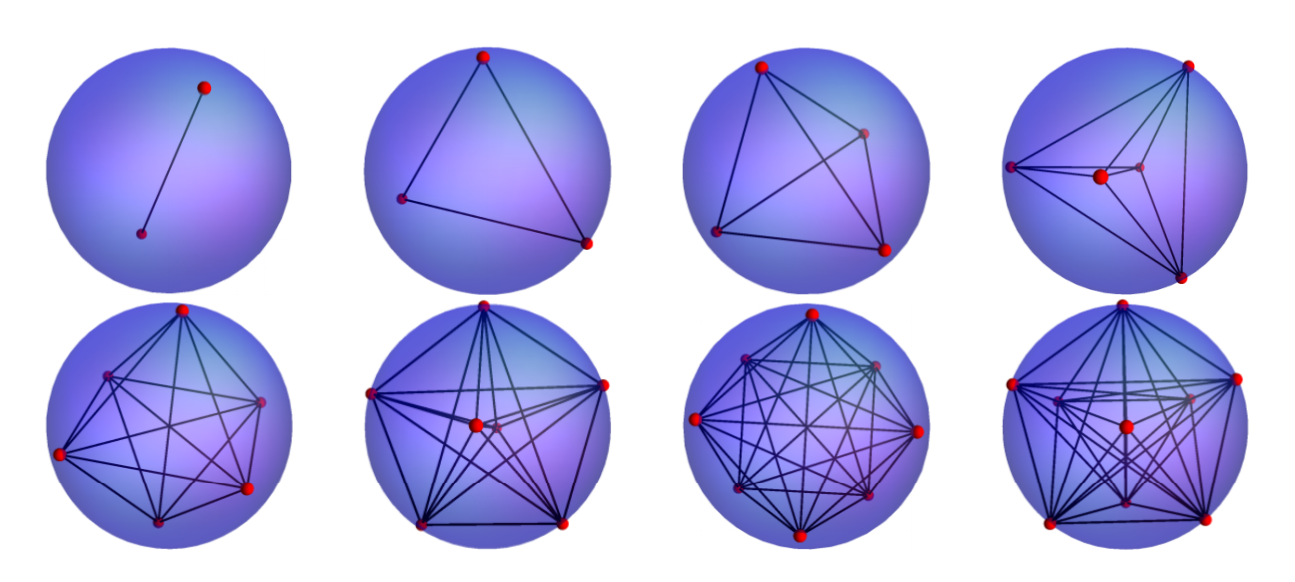## Exact energy computation of the one component plasma on a sphere for even values of the coupling parameter

#### Journal of Statistical Physics 164, 969 (2016)

The two dimensional one component plasma 2dOCP is a classical system consisting of N identical particles with the same charge q confined in a two dimensional surface with a neutralizing background. The Boltzmann factor at temperature T may be expressed as a Vandermonde determinant to the power Γ=q2/(kBT). Several statistical properties of the 2dOCP have been studied by expanding the Boltzmann factor in the monomial basis for even values of Γ. In this work, we use this formalism to compute the energy of the 2dOCP on a sphere. Using the same approach the entropy is computed. The entropy as well as the free energy in the thermodynamic limit have a universal finite-size correction term (χ/12) log N, where χ=2 is the Euler characteristic of the sphere. A non-recursive formula for coefficients of monomial functions expansion is used for exploring the energy as well as structural properties for sufficiently large values of Γ to appreciate the crystallization features for N=2,3,…,9 particles. Finally, we make a brief comparison between the exact and numerical energies obtained with the Metropolis method for even values of Γ.

`Universidad de los Andes | Vigilada MineducaciónReconocimiento como Universidad: Decreto 1297 del 30 de mayo de 1964.Reconocimiento personería jurídica: Resolución 28 del 23 de febrero de 1949 Minjusticia.`